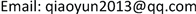1. 引言

2. 定义

1) 论证形式定义 

2) 论证形式有效性定义 

3. 论证形式有效性判断方法

1) 用真值表判断

2) 用反证法判断

3) 用重言式判断

4) 用推理规则判断

i) p ∨ q 前提引入

ii) p → r 前提引入

iii) q → r 前提引入

iv) r (1)(2)(3)构造性两难

v) q 前提引入

vi) q ∧ r 合取式

4. 论证形式有效性算法

import java.lang.Math;

public class love {

public static int YH(int p, int q){

if(p == 1 & q == 0){

return 0;}

else{return 1;}}//函数YH为蕴含函数定义；

public static int Y(int array[][],int n,int m){

int cou = 1;int geshu = 0;

int p = 0, q = 0,r = 0, s = 0, t = 0, u = 0;

for(int i = 0; i < n; i ++){

for(int j = 0;j <= m; j ++){

switch(j){

case 0:

p = array[i][j];

break;

case 1:

q = array[i][j];

break;

case 2:

r = array[i][j];

break;

case 3:

s = array[i][j];

break;

case 4:

t = array[i][j];

break;

case 5:

u = array[i][j];//变元不够时可再进行添加；

default:

break;}}

int y = YH(YH(p|q,r)&YH(r,s|t)&YH(t,u)&((~s)&(~u)),((~p)&(q)));//需要验证的论证形式改写用第三种方法写出的语句形式；

array[i] = y;

if(y == 0) cou = 0;}

for(int x = 0; x < array.length / 2; x ++){

int temp = array[x];

array[x] = array[array.length-1-x];

array[array.length-1-x] = temp;}

for(int i = 0; i < array.length; i ++){

if(array[i]==0){

System.out.print(\n此论证形式无效。\n);break;}//只要遇到赋值时结果为0，此语句形式不是重言式，此论证形式无效；

else{geshu ++;}}

if(geshu==n){System.out.print(\n此论证形式有效。\n);}//所有赋值结果都为1时，此语句为重言式，论证形式有效；

return 0;}

public static void main(String args[]){

String str = YH(YH(p|q,r)&YH(r,s|t)&YH(t,u)&((~s)&(~u)),((~p)&(q)))//与int y保持一致；

char[] cha1 = new char[str.length()];

char[] cha2 = new char[str.length()];

for(int i =0; i < str.length(); i ++){

cha1[i] = str.charAt(i);}

int count = 0;

for(int j = 0; j < str.length(); j ++){

if(((int)(cha1[j]) >= 97 & (int)(cha1[j]) <= 122)){

for(int k = j+1; k < str.length(); k ++){

if(cha1[j] == cha1[k]){

cha2[j] = cha1[j];}}}//计算小写字母个数；

else{

if(((int)(cha1[j]) > 122)| ((int)(cha1[j]) < 97)){

cha2[j] = cha1[j];}}}//计算其它字符个数；

for(int i =0; i < cha2.length; i ++){

if((int)(cha2[i]) != 0){

count ++;} }//计算重复的小写字母个数；

count = (int) (str.length()) - count;//计算输入的语句形式有几个变元；

//System.out.print(\n此论证形式变元个数为：+count+\n);此处可输出；

int[][] cha3 = new int[(int)(Math.pow(2,count))][count+1];

for(int i = (int)(Math.pow(2,count))-1; i >= 0; i --){

int s = i;

int t = i;

while(s != 0){

for(int j = 0;j < count;j ++){

cha3[t][j] = s % 2;

s = s / 2;}}}//采用变元赋值时每次十进制多1的方法，将十进制改写成2进制形式给变元赋值；

//System.out.print( +cha3[t][j]);//输出每个变元的赋值；可输出

//System.out.println();}}

for(int j = 0;j < count;j ++){

cha3[j] = 0;}

//System.out.print( +cha3[j]);//输出每个变元的赋值；可输出

System.out.println();

int y= Y(cha3,(int)(Math.pow(2,count)),count+1);} }//调用Y函数计算最后结果。